Definitions of Square Dance Calls and Concepts

Index -->  Plus  |  A1  |  A2  |  C1  |  C2  |  C3A  |  C3B  |  C4  |  NOL  |
Definitions (Text Only) -->  Plus  |  A1  |  A2  |  C1  |  C2  |  C3A  |  C3B  |  C4  |  NOL  |
 Find call:

2 x 4 \$B\$+\$i(B.

Centers \$B\$O(B Phantom Columns Circulate twice \$B\$r\$7(B, Ends \$B\$O(B Split Circulate twice \$B\$r\$7\$^\$9(B.

\$BJ?9T\$J(B Line \$B\$^\$?\$O(B Column \$B\$+\$i\$O(B Column \$B\$G=*\$o\$j(B; T-Bone \$B\$+\$i\$OMM!9\$J(B formation \$B\$G=*\$o\$j\$^\$9(B.

Change Your Image \$B\$O(B 2 \$B%Q!<%H\$N%3!<%k\$G(B, 4 \$B%Q!<%H\$KJ,\$1\$k\$3\$H\$b\$G\$-\$^\$9(B.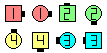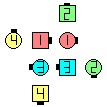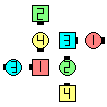Change Your Image\$B\$NA0(B 1/2\$B\$N8e(B (\$B=*\$o\$j(B)

\$BCm
• \$BDL\$j\$9\$.\$NLdBj\$rHr\$1\$k\$?\$a\$K(B, Ends \$B\$O(B centers \$B\$,>l=j\$r6u\$1\$k\$N\$rBT\$C\$F\$+\$iF0\$\$\$?J}\$,\$h\$\$\$3\$H\$,\$"\$j\$^\$9(B.
• Change Your Image 1 & 1/2 \$B\$^\$?\$O(B twice \$B\$G\$O(B, \$B;O\$a\$N(B Change Your Image \$B\$N8e\$K(B ends \$B\$H(B centers \$B\$r(B Re-evaluate \$B\$9\$k(B (\$B:FI>2A\$9\$k(B, \$B8+D>\$9(B) \$B\$3\$H\$KCm0U\$7\$F\$/\$@\$5\$\$(B.
• \$BJ?9T\$J(B Line \$B\$+\$i\$O(B, Change Your Image \$B\$O(B shape-changer \$B\$G\$9(B (\$B\$9\$J\$o\$A(B, \$B;O\$a\$N(B spot \$B\$+\$i(B 90° \$B2s\$C\$?(B column \$B\$H\$J\$j\$^\$9(B). Column \$B\$+\$i\$O(B, Change Your Image \$B\$O(B shape-changer \$B\$G\$O\$"\$j\$^\$;\$s(B.
• Magic Change Your Image [C3BV]: 2 x 4 \$B\$N(B ends \$B\$,0lHLE*\$J(B column \$B\$+\$i(B. Centers \$B\$O(B Phantom Columns Magic Column Circulate twice \$B\$r\$7(B, ends \$B\$O(B Magic Column Split Circulate twice \$B\$r\$7\$^\$9(B.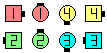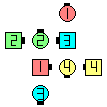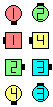Magic Change Your Image\$B\$NA0(B 1/2\$B\$N8e(B (\$B=*\$o\$j(B)Choreography for Change Your ImageComments? Questions? Suggestions?

https://www.ceder.net/def/changeyourimage.php?language=japan&level=master
10-August-2020 17:34:52